Area of Path and Cross roads - Worksheet

Chapter 9 Class 7 Perimeter and Area
Serial order wise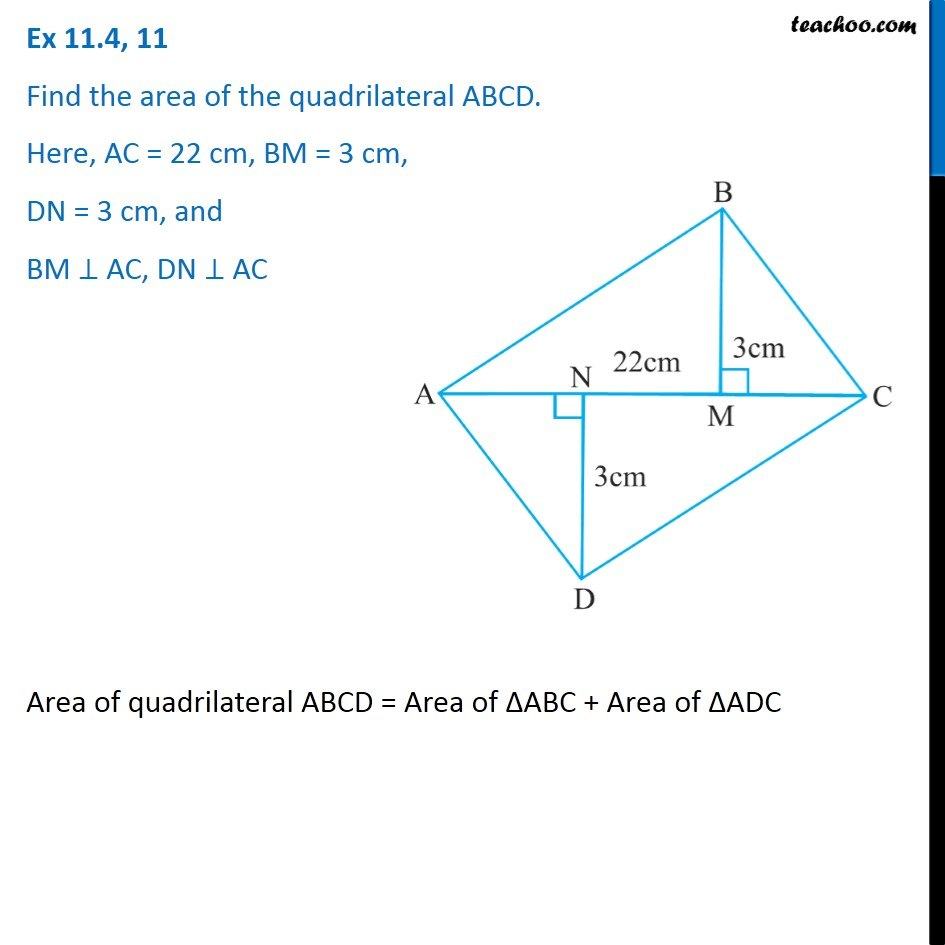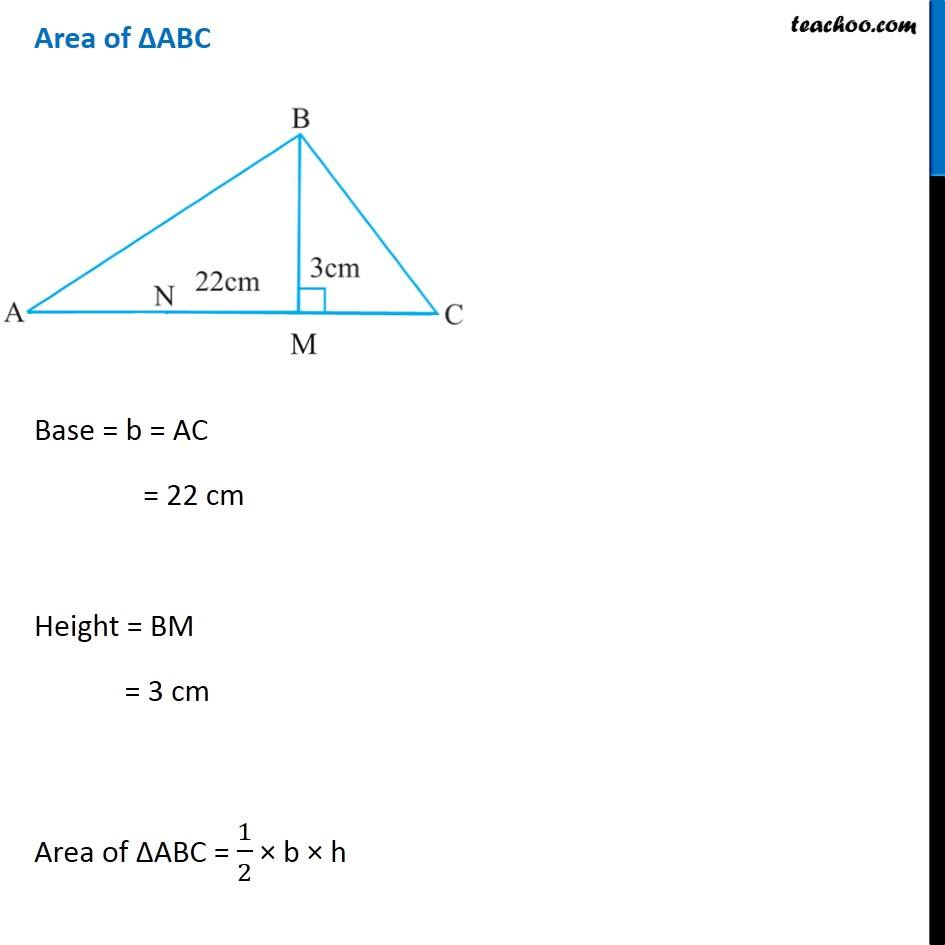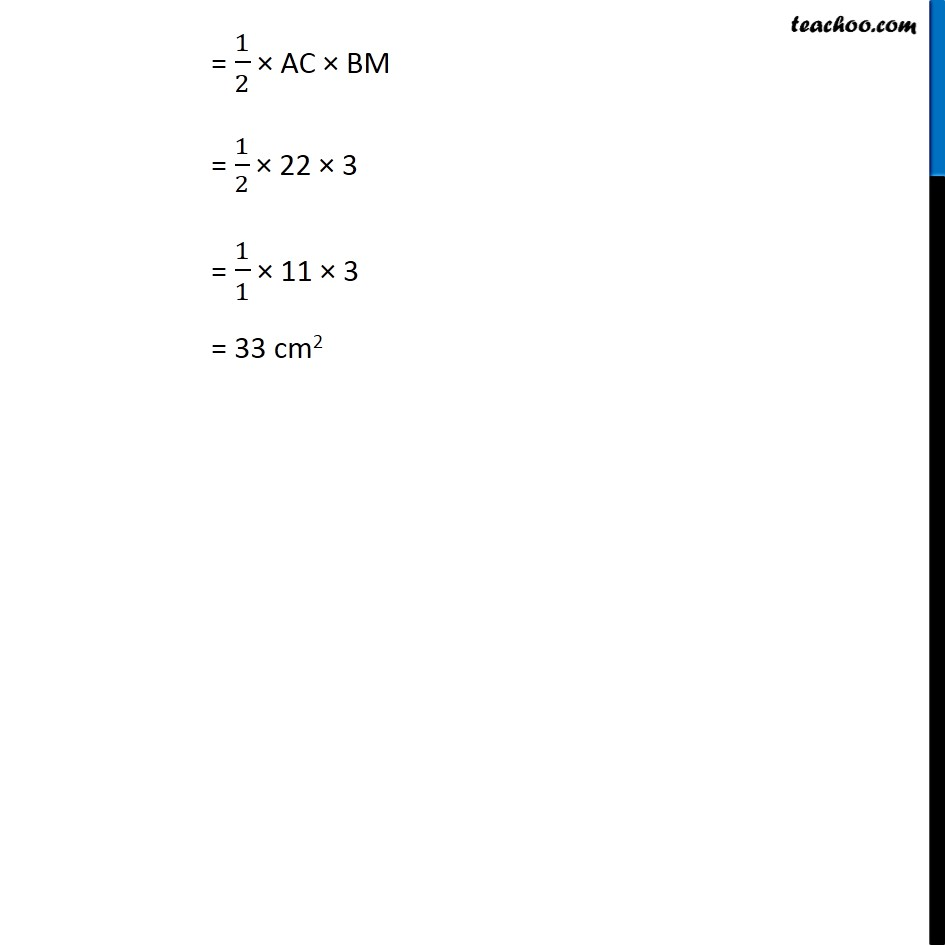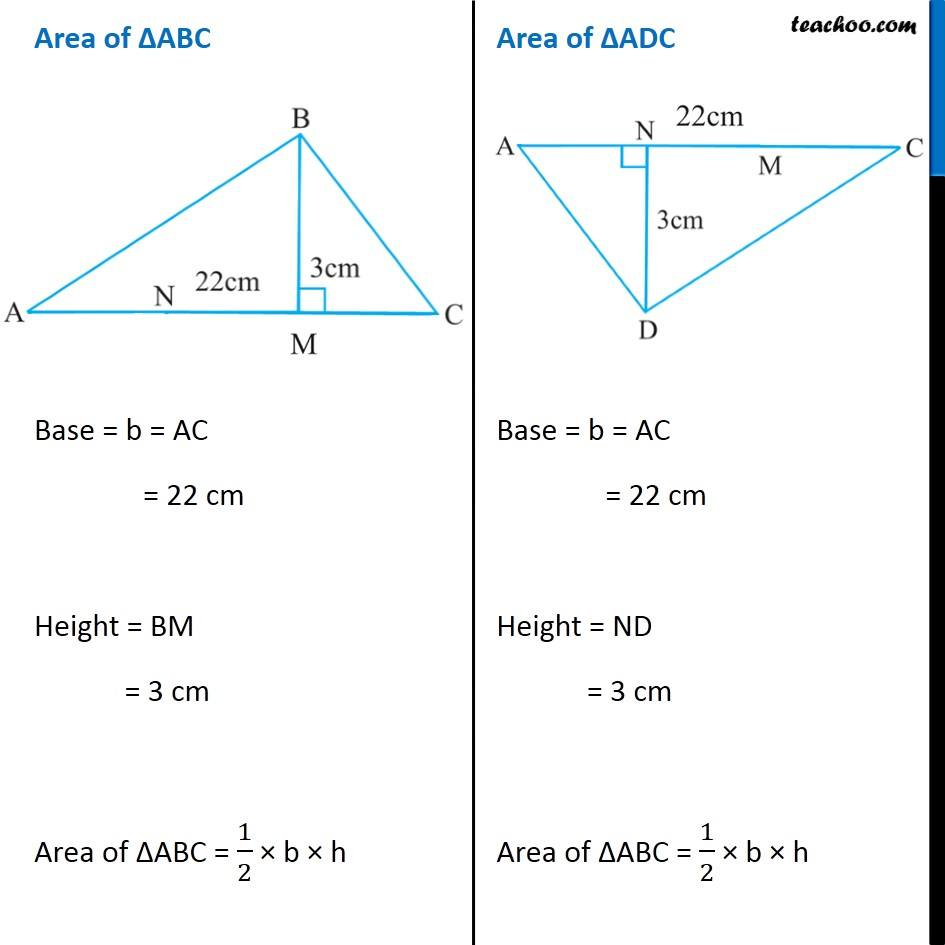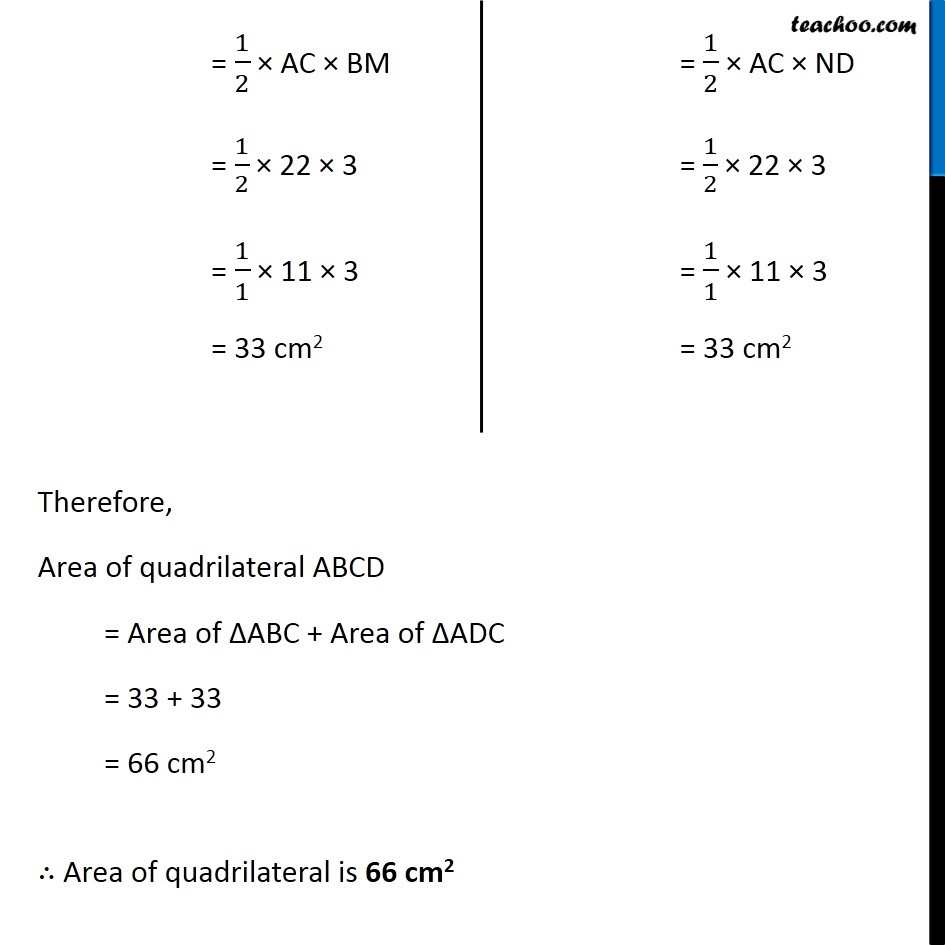Learn in your speed, with individual attention - Teachoo Maths 1-on-1 Class

### Transcript

Question 11 Find the area of the quadrilateral ABCD. Here, AC = 22 cm, BM = 3 cm, DN = 3 cm, and BM ⊥ AC, DN ⊥ AC Area of quadrilateral ABCD = Area of ∆ABC + Area of ∆ADC Area of ∆ABC Base = b = AC = 22 cm Height = BM = 3 cm Area of ∆ABC = 1/2 × b × h = 1/2 × AC × BM = 1/2 × 22 × 3 = 1/1 × 11 × 3 = 33 cm2 Area of ∆ABC Base = b = AC = 22 cm Height = BM = 3 cm Area of ∆ABC = 1/2 × b × h Area of ∆ADC Base = b = AC = 22 cm Height = ND = 3 cm Area of ∆ABC = 1/2 × b × h = 1/2 × AC × BM = 1/2 × 22 × 3 = 1/1 × 11 × 3 = 33 cm2 = 1/2 × AC × ND = 1/2 × 22 × 3 = 1/1 × 11 × 3 = 33 cm2 Therefore, Area of quadrilateral ABCD = Area of ∆ABC + Area of ∆ADC = 33 + 33 = 66 cm2 ∴ Area of quadrilateral is 66 cm2